Related Articles

# How to access different rows of a multidimensional NumPy array?

• Last Updated : 11 Oct, 2020

Let us see how to access different rows of a multidimensional array in NumPy. Sometimes we need to access different rows of multidimensional NumPy array-like first row, the last two rows, and even the middle two rows, etc. In NumPy , it is very easy to access any rows of a multidimensional array. All we need to do is Slicing the array according to the given conditions. Whenever we need to perform analysis, slicing plays an important role.

Case 1: In 2-Dimensional arrays

Attention geek! Strengthen your foundations with the Python Programming Foundation Course and learn the basics.

To begin with, your interview preparations Enhance your Data Structures concepts with the Python DS Course. And to begin with your Machine Learning Journey, join the Machine Learning - Basic Level Course

Example 1: Accessing the First and Last row of a 2-D NumPy array

## Python3

 `# Importing Numpy module``import` `numpy as np`` ` `# Creating a 3X3 2-D Numpy array``arr ``=` `np.array([[``10``, ``20``, ``30``], ``                ``[``40``, ``5``, ``66``], ``                ``[``70``, ``88``, ``94``]])`` ` `print``(``"Given Array :"``)``print``(arr)`` ` `# Access the First and Last rows of array``res_arr ``=` `arr[[``0``,``2``]]``print``(``"\nAccessed Rows :"``)``print``(res_arr)`

Output: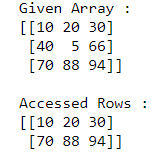In the above example, we access and print the First and Last rows of the 3X3 NumPy array.

Example 2: Accessing the Middle row of 2-D NumPy array

## Python3

 `# Importing Numpy module``import` `numpy as np`` ` `# Creating a 3X4 2-D Numpy array``arr ``=` `np.array([[``101``, ``20``, ``3``, ``10``], ``                ``[``40``, ``5``, ``66``, ``7``], ``                ``[``70``, ``88``, ``9``, ``141``]])``                ` `print``(``"Given Array :"``)``print``(arr)`` ` `# Access the Middle row of array``res_arr ``=` `arr[``1``]``print``(``"\nAccessed Row :"``)``print``(res_arr)`

Output: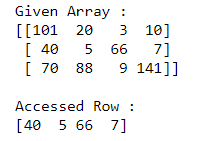In the above example, we access and print the Middle row of the 3X4 NumPy array.

Example 3: Accessing the Last three rows of 2-D NuNumPy py array

## Python3

 `# Importing Numpy module``import` `numpy as np`` ` `# Creating a 4X4 2-D Numpy array``arr ``=` `np.array([[``1``, ``20``, ``3``, ``1``], ``                ``[``40``, ``5``, ``66``, ``7``], ``                ``[``70``, ``88``, ``9``, ``11``],``               ``[``80``, ``100``, ``50``, ``77``]])`` ` `print``(``"Given Array :"``)``print``(arr)`` ` `# Access the Last three rows of array``res_arr ``=` `arr[[``1``,``2``,``3``]]``print``(``"\nAccessed Rows :"``)``print``(res_arr)`

Output: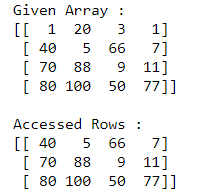In the above example, we access and print the last three rows of the 4X4 NumPy array.

Example 4: Accessing the First two rows of a 2-D NumPy array

## Python3

 `# Importing Numpy module``import` `numpy as np`` ` `# Creating a 5X4 2-D Numpy array``arr ``=` `np.array([[``1``, ``20``, ``3``, ``1``], ``                ``[``40``, ``5``, ``66``, ``7``], ``                ``[``70``, ``88``, ``9``, ``11``],``               ``[``80``, ``100``, ``50``, ``77``],``               ``[``1``, ``8.5``, ``7.9``, ``4.8``]])`` ` `print``(``"Given Array :"``)``print``(arr)`` ` `# Access the First two rows of array``res_arr ``=` `arr[[``0``,``1``]]``print``(``"\nAccessed Rows :"``)``print``(res_arr)`

Output: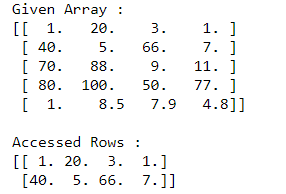In the above example, we access and print the First two rows of the 5X4 NumPy array.

Case 2: In 3-Dimensional arrays

Example 1: Accessing the Middle rows of 3-D NumPy array

## Python3

 `# Importing Numpy module ``import` `numpy as np`` ` `# Creating 3-D Numpy array``n_arr ``=` `np.array([[[``10``, ``25``, ``70``], [``30``, ``45``, ``55``], [``20``, ``45``, ``7``]], ``                  ``[[``50``, ``65``, ``8``], [``70``, ``85``, ``10``], [``11``, ``22``, ``33``]]])`` ` `print``(``"Given 3-D Array:"``)``print``(n_arr)`` ` `# Access the Middle rows of 3-D array``res_arr ``=` `n_arr[:,[``1``]]``print``(``"\nAccessed Rows :"``)``print``(res_arr)`

Output: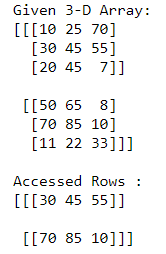In the above example, we access and print the Middle rows of the 3-D NumPy array.

Example 2: Accessing the First and Last rows of 3-D NumPy array

## Python3

 `# Importing Numpy module ``import` `numpy as np`` ` `# Creating 3-D Numpy array``n_arr ``=` `np.array([[[``10``, ``25``, ``70``], [``30``, ``45``, ``55``], [``20``, ``45``, ``7``]], ``                  ``[[``50``, ``65``, ``8``], [``70``, ``85``, ``10``], [``11``, ``22``, ``33``]],``                 ``[[``19``, ``69``, ``36``], [``1``, ``5``, ``24``], [``4``, ``20``, ``96``]]])`` ` ` ` `print``(``"Given 3-D Array:"``)``print``(n_arr)`` ` `# Access the First and Last rows of 3-D array``res_arr ``=` `n_arr[:,[``0``, ``2``]]``print``(``"\nAccessed Rows :"``)``print``(res_arr)`

Output: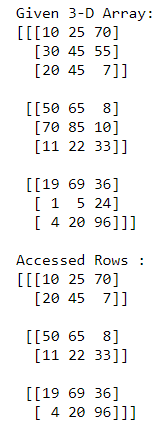In the above example, we access and print the First and Last rows of the 3-D NumPy array.

My Personal Notes arrow_drop_up